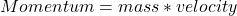## A 10 kg firework is shot in the air at 75 m/s. What is the momentum of the firework?

Question

A 10 kg firework is shot in the air at 75 m/s. What is the momentum of the firework?

in progress 0
6 months 2021-08-22T14:56:25+00:00 1 Answers 6 views 0

## Answers ( )

Momentum = 750 Kgm/s

Explanation:

Given the following data;

Mass = 10 kg

Velocity = 75 m/s

To find the momentum;

Momentum can be defined as the multiplication (product) of the mass possessed by an object and its velocity. Momentum is considered to be a vector quantity because it has both magnitude and direction.

Mathematically, momentum is given by the formula;Substituting into the formula, we have;

Momentum = 10 * 75

Momentum = 750 Kgm/s